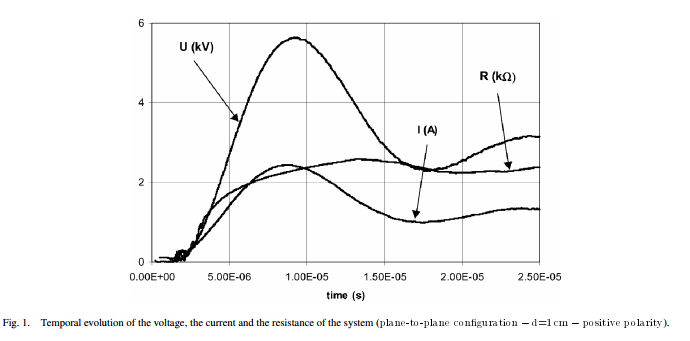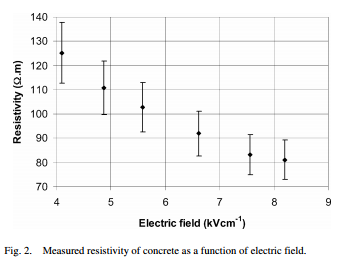# Electrical Parameters Associated With Discharges in Resistive Soils

## 2. 土壤电阻率的测定

• 其中石英占82.8% ，钾长石占2.9% ，石灰石占7.2% ，碳酸钙占3.1% ，云母占0.9% ，黑云母占2.4% ，磁铁矿占0.7% ;
• 黑色粘土;
• 由砂(50%)和粘土(50%)制成的混合物;
• 混凝土由$4.8dm^3$砂土，$1.6dm^3$水泥和$1dm^3$水组成。

### 2.1 均匀电场法$I = Ir + Ic = Ir + C \frac { d U } { d t } \tag{1}$

$R _ { t } = \rho \times \frac { d } { S } \tag{2}$

$\rho = 1600 \Omega \cdot m$